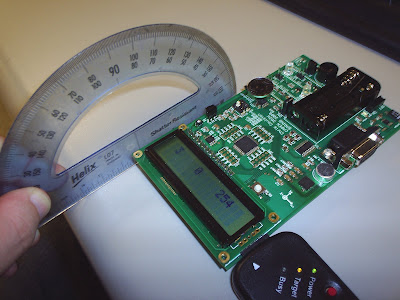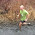Monday, April 13, 2009

Accelerometer experimental values

The following test was performed on the calibrated output of the accelerometer. Initially the accelerometer was calibrated in the static state sitting on a table; increments based on a degree measurement. The X and Z were measured as shown in Figure 1, and Y was measured as shown in Figure 2.

Calibration of the module is done by taking a snapshot of the x, y, and z values during startup. The values in Table 1 are simply the difference between these initial values and the sampled values at the various angles. That is
K_calibrated = K_initial - K_sample,
where K is either the x, y, or z direction.

Note:
This experiment is going to be refined to show the sampled value along with the calibrated value, where the rollover is shown. Updates to come...Figure 1: Measurement setup for X and Z axisFigure 2: Measurement setup for the Y axis

It is noted in the test that the X and Y values correspond for their respective angles.

1 comment:

1.The relationship between the X and Y sensor angle and sensor output is unclear from your table. The small jump from 0 degrees to 15 degrees produces a large change in the sensor output. Furthermore, the change in sensor output seems to be on the low side. The ADC swing was around 0x200 from +1g to -1g (according to the lab3.hex file).

I am also unsure what you mean by "calibrate."